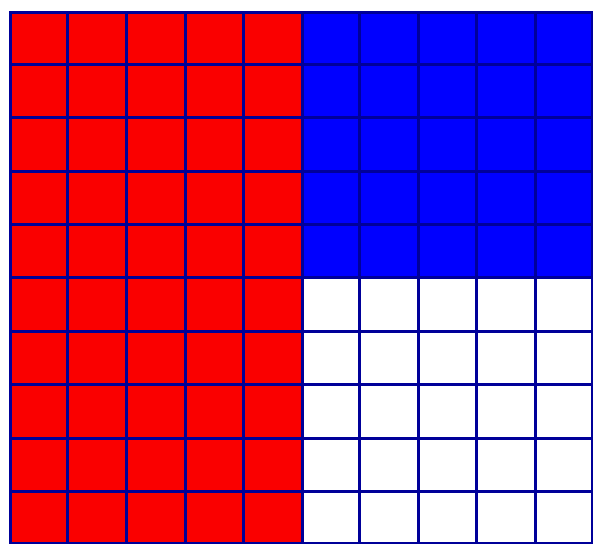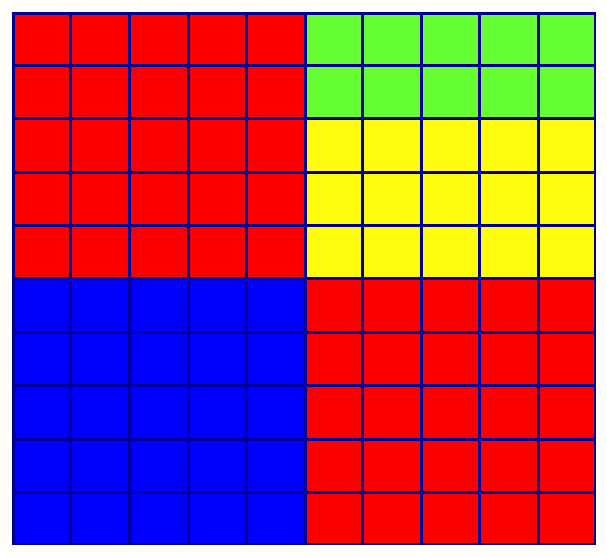# Percentages

## Percent means "per hundred" or "out of one hundred".

### We use a special symbol '%' to represent percent.

A good way of introducing percentages is to visualise them by using a 100 square and to colour in the percentages.

This square has 50 out of 100 squares coloured in red.

50 out of 100 is 50 percent ... 50% are red

note that 50 out of 100 are not coloured in

50 out of 100 is 50 percent ... 50% are not coloured

This square also has 50 out of 100 square coloured in red.

50 out of 100 is 50 percent ... 50% are red

It also has 25 out of 100 squares coloured in blue

25 out of 100 is 25 percent ... 25% are blue

note that 50 out of 100 are not coloured in

25 out of 100 is 25 percent ... 25% are not coloured

This square also has 50 out of 100 square coloured in red.

50 out of 100 is 50 percent ... 50% are red

It also has 25 out of 100 squares coloured in blue

25 out of 100 is 25 percent ... 25% are blue

10 out of 100 are green ... 10% are green

15 out of 100 are yellow ... 15% are yellowPrimary Resources have an excellent powerpoint resource - Percentages, Fractions of 100,
that is worth working through with your child. As is this Percentage Squares worksheet.

_________________________________

To find the percentage of a number we divide the total amount by 100 - this will tell us what 1% of the total is, and then multiply by the amount that we wish to know.

To find 10% of 100, divide 100 by 100 = 1, then 10 x 1 = 10 ... 10% of 100 is 10

To find 10% of 50, divide 50 by 100 = 0.5, then 10 x 0.5 = 5 ... 10% of 50 is 5
(remember that to divide by 100, shift the number 2 places to the right)

To find 15% of 300, divide 300 by 100 = 3, then 15 x 3 = 45 ... 15% of 300 is 45

___________________________________

Another method of finding the percentage of a number is to change the percentage required into a decimal and the multiply the number by it.

30% means 30 out of 10, which is a fraction 30/100 - to convert fractions to decimals, we divide the numerator by the denominator - 30 ÷ 100 = 0.3.

### To find the percentage of a number we can multiply by the equivalent decimal.

To find 10% of 100, decimal equivalent of 10% is 10 ÷ 100 = 0.1, 100 x 0.1 = 10
(remember that to multiply by 100, shift the number 2 places to the left)

To find 10% of 50, decimal equivalent of 10% is 10 ÷ 100 = 0.1, 50 x 0.1 = 5

To find 15% of 300, decimal equivalent of 15% is 15 ÷ 100 = 0.15, 300 x 0.15 = 45

_____________________________________

## Some percentages are worth remembering

50% means a half

25 % means a quarter

75 % means three quarters

10 % means one tenth

Try these worksheets from Primary
Resources:

Percentages 1

Percentages 2